## 5.13 I had this one in an exam.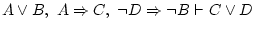In the final exam of ILO they were asking, and I needed a very very long time until I got it: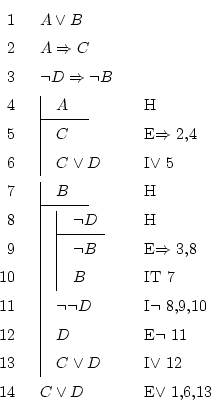Remark that the result we're searching,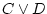, is a disjunction. Since you already know the disjunction introduction, you could simply search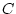, and then use that rule to get. Or if withdidn't work, you could try with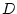, since ifis true, thenalso is, and we're done.

Unfortunately,is not always true, andalso isn't always true (on the other hand,is always true, and that's what we're trying to prove). After seeing this, we must search another method which works with the two formulas,and, at the same time, since it seems that if we take only one without looking at the other, then it does not provide much information.

To use the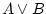we must use proof by cases. We will try to see that both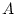and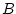lead to, since if we can do that, we will have finished.implies, and ifis true thenalso is, soimplies.

With, what we know doesn't relate it tobut to. We want. Hardly we will make truebecause of, so we will try to make true just the. To do so, we will use reduction to the absurd: suppose thatis false, then it holds that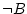thanks to the formula on line 3. But we were under the supposition thatwas true, so our hypothesis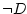can't be true, thusis true, and so is.

Sinceis true, and both paths lead to, we finally see thatis always true.

If you are skilled working with logical formulas, you will have seen that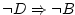is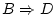. This simplifies the problem and helps understanding it faster. But anyway, you can't changetodirectly, you would have to do it step by step.

Daniel Clemente Laboreo 2005-05-17